# Problem 55370. Easy Sequences 69: Radix Economy of Factorials

The Radix Economy of a number in a particular base is the number of digits needed to express it in that base, multiplied by the base. In functional form we write: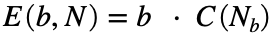, where E is the radix econony, b and N, are the base and number, respectively, and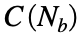, is the number of digits of the base-b representation of N.
For example, if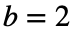and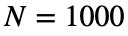, then the radix economy is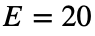:
>> E = 2 * length(dec2bin(1000))
>> E =
20
Given a base b, and an integer n, calculate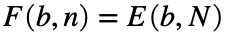, for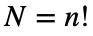.
For example, if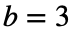and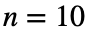, we have:
>> F = 3 * length(dec2base(factorial(10),3))
>> F =
42
----------------
NOTE: As it is, this problem is quite simple. In fact, a solution is already given for small values of b and n. Therefore, to make it a bit interesting, some built-in functions are disabled.

### Solution Stats

60.0% Correct | 40.0% Incorrect
Last Solution submitted on Sep 12, 2023

### Community Treasure Hunt

Find the treasures in MATLAB Central and discover how the community can help you!

Start Hunting!